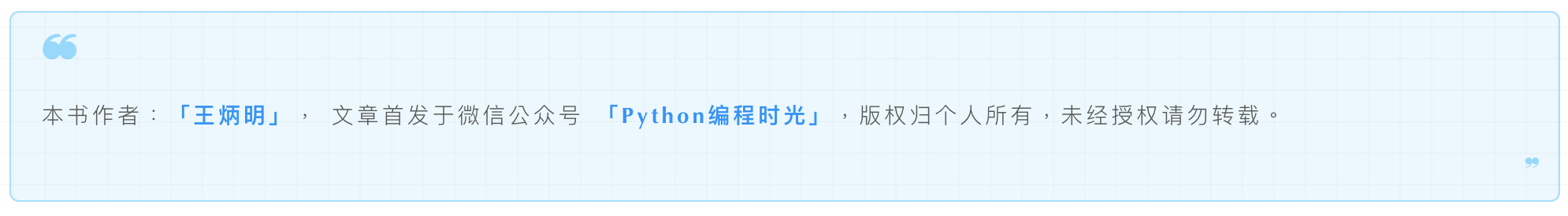# 1.30 break /continue 和 上下文管理器哪个优先级高？¶```import time
import contextlib

@contextlib.contextmanager
def runtime(value):
time.sleep(1)
print("start: a = " + str(value))
yield
print("end: a = " + str(value))

a = 0
while True:
a+=1
with runtime(a):
if a % 2 == 0:
break
```

```start: a = 1
end: a = 1
start: a = 2
end: a = 2
```

1. continue 与 break 一样，如果先遇到上下文管理器会先进行资源的释放

2. 上面只举例了 while 循环体，而 for 循环也是同样的。